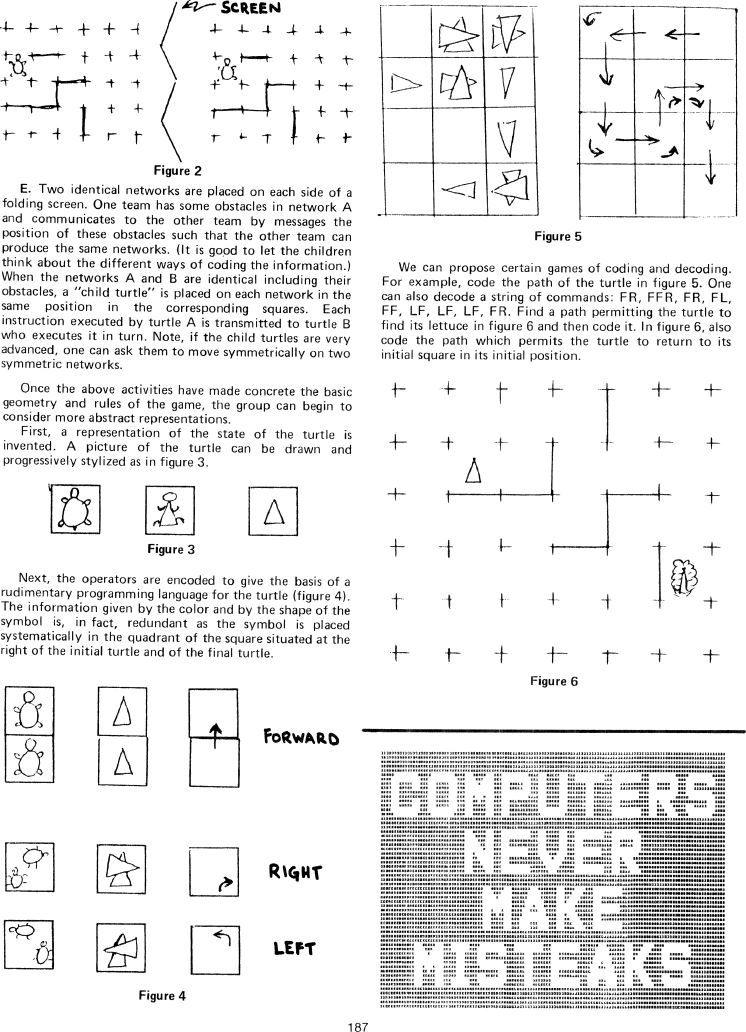The Best of Creative Computing Volume 1 (published 1976)

 `Page 187 << PREVIOUS >> NEXT Jump to page: Go to contents Go to thumbnails`

Turtle Geometry Without Hardware```[image]Figure2
E. Two identical networks are placed on each side of a
folding screen. One team has some obstacles in network A
and communicates to the other team by messages the
position of these obstacles such that the other team can
produce the same networks. (It is good to let the children
think about the different ways of coding the information.)
When the networks A and B are identical including their
obstacles, a "child turtle" is placed on each network in the
same position in the corresponding squares. Each
instruction executed by turtle A is transmitted to turtle B
who executes it in turn. Note, if the child turtles are very
advanced, one can ask them to move symmetrically on two
symmetric networks.

Once the above activities have made concrete the basic
geometry and rules of the game, the group can begin to
consider more abstract representations.

First, a representation of the state of the turtle is
invented. A picture of the turtle can be drawn and
progressively stylized as in figure 3.

Figure 3
Next, the operators are encoded to give the basis of a
rudimentary programming language for the turtle (figure 4).

The information given by the color and by the shape of the
symbol is, in fact, redundant as the symbol is placed
systematically in the quadrant of the square situated at the
right of the initial turtle and of the final turtle.

[image]Figure4
[image]Figure5
We can propose certain games of coding and decoding.

For example, code the path of the turtle in figure 5. One
can also decode a string of commands: FR, FFR, FR, FL,
FF, LF, LF, LF, FR. Find a path permitting the turtle to
find its lettuce in figure 6 and then code it. In figure 6, also
code the path which permits the turtle to return to its
initial square in its initial position.

[image]Figure 6
[image]Page 187        << PREVIOUS        >> NEXT        Jump to page:                Go to contents        Go to thumbnails

<!--
google_ad_client = "pub-0754410284344153";
google_ad_width = 728;
google_ad_height = 90;
google_ad_format = "728x90_as";
google_ad_type = "text_image";
google_ad_channel ="0446829006";
google_color_border = "FFFFFF";
google_color_bg = "FFFFFF";
google_color_link = "0000FF";
google_color_url = "666666";
google_color_text = "000000";
google_alternate_color = "FFFFFF";
//-->

```